# Tkinter Grid

Summary: in this tutorial, you’ll learn how to use the Tkinter grid geometry manager to position widgets on a window.

## Introduction to the Tkinter grid geometry manager

The grid geometry manager uses the concepts of rows and columns to arrange the widgets.

The following shows a grid that consists of four rows and three columns: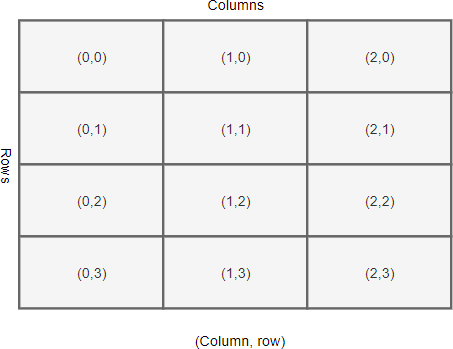Each row and column in the grid is identified by an index. By default, the first row has an index of zero, the second row has an index of one, and so on. Likewise, the columns in the grid have indexes of zero, one, two, etc.

The indexes of rows and columns in a grid don’t have to start at zero. In addition, the row and column indexes can have gaps.

For example, you can have a grid whose column indexes are 1, 2, 10, 11, and 12. This is useful when you plan to add more widgets in the middle of the grid later.

The intersection of a row and a column is called a cell. A cell is an area that you can place a widget. A cell can hold only one widget. If you place two widgets in a cell, they’ll be on top of each other.

To place multiple widgets in a cell, you use a `Frame` or `LabelFrame` to wrap the widgets and place the `Frame` or `LabelFrame` on the cell.

The width of a column depends on the width of the widget it contains. Similarly, the height of a row depends on the height of the widgets contained within the row.

Rows and columns can span. The following illustrates a grid that has the cell (1,1) that spans two columns and the cell (0,2) that spans two rows: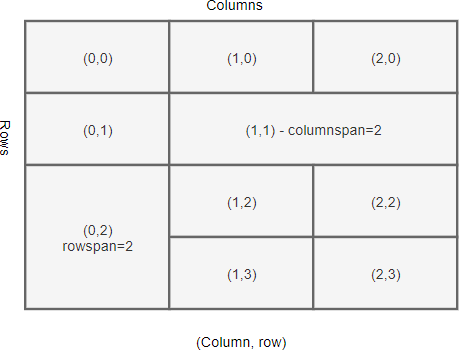## Setting up the grid

Before positioning widgets on a grid, you’ll need to configure the rows and columns of the grid.

Tkinter provides you with two methods for configuring grid rows and columns:

```
```

`container.columnconfigure(index, weight) container.rowconfigure(index, weight)`

Code language: CSS (css)

The `columnconfigure()` method configures the column `index` of a grid.

The `weight` determines how wide the column will occupy, which is relative to other columns.

For example, a column with a weight of 2 will be twice as wide as a column with a weight of 1.

## Positioning a widget on the grid

To place a widget on the grid, you use the widget’s `grid()` method:

```
```

`widget.grid(**options)`

Code language: Python (python)

The `grid()` method has the following parameters:

Parameters Meaning
column The column index where you want to place the widget.
row The row index where you want to place the widget.
rowspan Set the number of adjacent rows that the widget can span.
columnspan Set the number of adjacent columns that the widget can span.
sticky If the cell is large than the widget, the `stick` option specifies which side the widget should stick to and how to distribute any extra space within the cell that is not taken up by the widget at its original size.

### Sticky

By default, when a cell is larger than the widget it contains, the grid geometry manager places the widget at the center of the cell horizontally and vertically.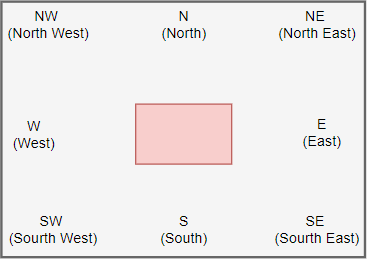To change this default behavior, you can use the `sticky` option. The sticky option specifies which edge of the cell the widget should stick to.

The value of the `sticky` has the following valid values:

Sticky Description
N North or Top Center
S South or Bottom Center
E East or Right Center
W West or Left Center
NW North West or Top Left
NE North East or Top Right
SE South East or Bottom Right
SW South West or Bottom Left
NS NS stretches the widget vertically. However, it leaves the widget centered horizontally.
EW EW stretches the widget horizontally. However, it leaves the widget centered vertically.

If you want to position the widget in the corner of the cell, you can use the N, S, E, and W.

The following example illustrates how to position a widget with the sticky option set to `N`: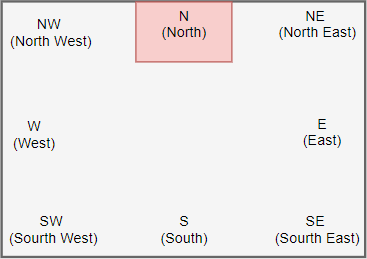If you want to position the widget centered against one side of the cell, you can use the NW (top left), NE (top right), SE (bottom right), and SW (bottom left).

The following example shows how to position a widget with the sticky option set to `NW`: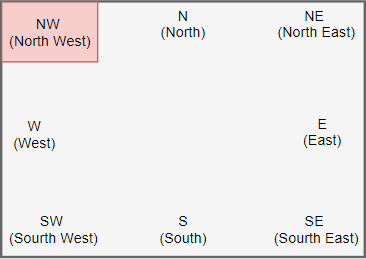NS stretches the widget vertically. However, it leaves the widget centered horizontally: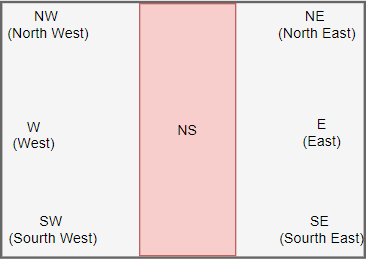EW stretches the widget horizontally. However, it leaves the widget centered vertically: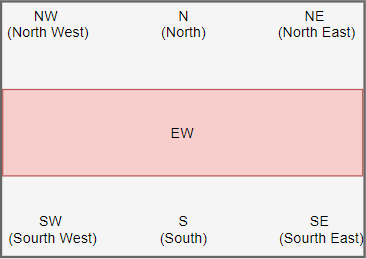To add paddings between cells of a grid, you use the `padx` and `pady` options. The `padx` and `pady` are external paddings:

```
```

`grid(column, row, sticky, padx, pady)`

Code language: Python (python)

To add paddings within a widget itself, you use `ipadx` and `ipady` options. The `ipadx` and `ipady` are internal paddings:

```
```

`grid(column, row, sticky, padx, pady, ipadx, ipady)`

Code language: Python (python)

The internal and external paddings default to zero.

## Tkinter grid geometry manager example

In this example, we’ll use the grid geometry manager to design a login screen as follows: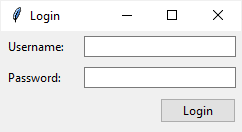The login screen uses a grid that has two columns and three rows. Also, the second column is three times as wide as the first column: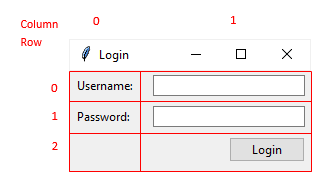The following shows the complete login window:

```
```

`import tkinter as tk from tkinter import ttk # root window root = tk.Tk() root.geometry("240x100") root.title('Login') root.resizable(0, 0) # configure the grid root.columnconfigure(0, weight=1) root.columnconfigure(1, weight=3) # username username_label = ttk.Label(root, text="Username:") username_label.grid(column=0, row=0, sticky=tk.W, padx=5, pady=5) username_entry = ttk.Entry(root) username_entry.grid(column=1, row=0, sticky=tk.E, padx=5, pady=5) # password password_label = ttk.Label(root, text="Password:") password_label.grid(column=0, row=1, sticky=tk.W, padx=5, pady=5) password_entry = ttk.Entry(root, show="*") password_entry.grid(column=1, row=1, sticky=tk.E, padx=5, pady=5) # login button login_button = ttk.Button(root, text="Login") login_button.grid(column=1, row=3, sticky=tk.E, padx=5, pady=5) root.mainloop() `

Code language: Python (python)

How it works.

First, use the `columnconfigure()` method to set the weight of the first and second columns of the grid. The width of the second column is three times bigger than the width of the first column.

```
```

`root.columnconfigure(0, weight=1) root.columnconfigure(1, weight=3)`

Code language: Python (python)

Second, position the username label on the first column and the username entry on the second column of the first row of the grid:

```
```

`username_label = ttk.Label(root, text="Username:") username_label.grid(column=0, row=0, sticky=tk.W, padx=5, pady=5) username_entry = ttk.Entry(root, textvariable=username) username_entry.grid(column=1, row=0, sticky=tk.E, padx=5, pady=5`

Code language: Python (python)

Third, position the password label and entry on the first and second column of the second row:

```
```

`password_label = ttk.Label(root, text="Password:") password_label.grid(column=0, row=1, sticky=tk.W, padx=5, pady=5) password_entry = ttk.Entry(root, textvariable=password, show="*") password_entry.grid(column=1, row=1, sticky=tk.E, padx=5, pady=5)`

Code language: Python (python)

Finally, position the login button on the third row. Since its `sticky` option sets to `E`, the button is aligned to the right of the third row.

```
```

`login_button = ttk.Button(root, text="Login") login_button.grid(column=1, row=3, sticky=tk.E, padx=5, pady=5)`

Code language: Python (python)

The following shows the same program. However, it uses object-oriented programming instead:

```
```

`import tkinter as tk from tkinter import ttk class App(tk.Tk): def __init__(self): super().__init__() self.geometry("240x100") self.title('Login') self.resizable(0, 0) # configure the grid self.columnconfigure(0, weight=1) self.columnconfigure(1, weight=3) self.create_widgets() def create_widgets(self): # username username_label = ttk.Label(self, text="Username:") username_label.grid(column=0, row=0, sticky=tk.W, padx=5, pady=5) username_entry = ttk.Entry(self) username_entry.grid(column=1, row=0, sticky=tk.E, padx=5, pady=5) # password password_label = ttk.Label(self, text="Password:") password_label.grid(column=0, row=1, sticky=tk.W, padx=5, pady=5) password_entry = ttk.Entry(self, show="*") password_entry.grid(column=1, row=1, sticky=tk.E, padx=5, pady=5) # login button login_button = ttk.Button(self, text="Login") login_button.grid(column=1, row=3, sticky=tk.E, padx=5, pady=5) if __name__ == "__main__": app = App() app.mainloop() `

Code language: Python (python)

## Summary

• Use the `columnconfigure()` and `rowconfigure()` methods to specify the weight of a column and a row of a grid.
• Use `grid()` method to position a widget on a grid.
• Use `sticky` option to align the position of the widget on a cell and define how the widget will be stretched.
• Use `ipadx``ipady` and `padx``pady` to add internal and external paddings.